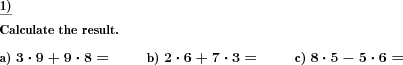Custom math worksheets at your fingertips# Details for problem "Add and subtract times table product"

Quickname: 7300

Elementary School, Primary School, Middle School.

## Summary

Addition and subtraction of times table products

## Example## Description

A great way to help children understand how to learn the order of operations is to use this problem that provides questions for children to solve and use their thinking skills.

In each problem, two products have to be added or subtracted. All numbers are taken from the times tables one to ten.

These problems can be used for different grades to achieve several learners’ outcomes such as:

• To introduce orders of operations in grade 3 to grade 5.
• To quickly recall the order of operations with numbers before introducing this concept in algebra.

This activity can be used to practice the skills to add and subtract times table product in groups, independent, or homework activity. Following are some instructional goals that can be achieved by exercising these worksheets.

1. Addition and subtraction concepts will be revised.
2. Apply times table to calculate products.
3. Learn to follow a sequence of steps to perform a particular task.
4. Practice for order of operations (PEMDAS/BEDMAS/BODMAS rules).
5. Essential skills to learn before learning algebraic arithmetic operations.

The number of problems and the number range to be worked on can be chosen. Operators can be chosen to be addition only, subtraction only or a mix of both. The answer key will give the solution to each problem in two steps by first solving products using the times table and then applying addition or subtraction.

The above acronyms for the rules of precedence expand as follows for different countries:

PEMDAS
BEDMAS
BODMAS

Download free printable worksheets for this math problem here. The worksheet contains the problems only, the solution sheet includes the answers. Just click on the respective link.

•Worksheet 1Solution sheet with answers
•Worksheet 2Solution sheet with answers
•Worksheet 3Solution sheet with answers

If you can not see the solution sheets for download, they may be filtered out by an ad blocker that you may have installed. If this is the case, please allow ads for this page and reload the page. The solution sheets will then reappear.

• Do these sample worksheets do not really fit?
• Do you need more math worksheets, with a different level of difficulty?
• Would you like to combine different problems on a worksheet and adjust them to your needs?
• As a teacher, you can put together your own worksheets using the automatically generated math problems provided.
With a free initial credit, you can start creating your own math worksheets in a few minutes.

You can try it for free! Register here, to create custom worksheets now!

## Customization options for this problem

Parameter
Possible values
Number of problems
1, 2, 3, 4, 5, 6, 7, 8, 9, 10

## Similar problems

Remark
Description
A similar problem covering the times table of only a single number
Addition and subtraction of times table products for a single number one to ten